Euclidean norm

(diff) ← Older revision | Latest revision (diff) | Newer revision → (diff)

Definition

Let$R$ be a commutative unital ring. A Euclidean norm on$R$ is a function$N$ from the set of nonzero elements of$R$ to the set of nonnegative integers, such for that for any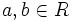$a,b \in R$ with$b$ not zero, there exist$q,r \in R$ such that: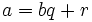$a = bq + r$

and either$r = 0$ or$N(r) < N(b)$. Such a pair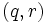$(q,r)$ is termed a quotient-remainder pair for$(a,b)$.$a$ here is the dividend and$b$ is the divisor.

For convenience, we set the norm of zero as$\infty$.

Note that we often assume the underlying commutative unital ring to be an integral domain.

A ring which admits a Euclidean norm is termed a Euclidean ring, and an integral domain which admits a Euclidean norm is termed a Euclidean domain.

Facts

The following can be readily verified for a Euclidean norm:

• If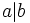$a|b$, then the norm of$b$ is at least as much as the norm of$a$.
• The units have the lowest possible Euclidean norm.

Kinds of Euclidean norms

Multiplicative Euclidean norm

Further information: multiplicative Euclidean norm

A Euclidean norm is multiplicative if for any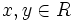$x,y \in R$,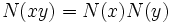$N(xy) = N(x)N(y)$.

An example of a multiplicative Euclidean norm is the usual absolute value function on the ring of integers.